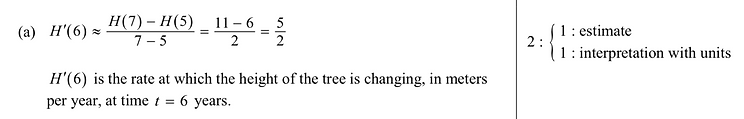top of page

## Unit 1 - Day 1

##### All Units
###### ​Learning Objectives
• Interpret the rate of change at an instant in terms of average rates of change over intervals containing that instant.

###### ​Success Criteria
• I can calculate and interpret an average rate of change.

• I can explain the difference between average and instantaneous rates of change.

• I can explain why new methods are needed to calculate change at an instant.

###### Quick Lesson Plan### Unit 1 Day 1 Video

Play Video
###### Overview

​Today’s lesson is an introduction into the world of calculus with a big question: how can we measure change at a single instant? The answer to this question will take several weeks to answer but today’s lesson highlights that there are in fact two kinds of rates of change: average and instantaneous. We will look at a car display to investigate why there are two measures of fuel efficiency and what each of these means. Students should be able to explain verbally the difference between change over an interval and change at an instant, and they should be able to calculate average rates of change using the slope formula. We will then explore how change at an instant gets us into some complicated math territory. The change in y and the change in x at any one particular instant is 0. So we have a slope of 0/0?? How can this be? Informally, we will introduce the idea that since we can’t actually divide by 0 we must let the change in y and change in x get really, really close to 0. This is the language of limits and it is the foundation of the rest of the course.​

##### ​
###### Teaching Tips

Although today’s lesson gets at a big idea, it is not necessary that students have a solid, operational understanding of limits by the end. We recommend not using formal limit notation until tomorrow though it’s worthwhile to use the language of “getting really, really close to ___” and “taking a limit” multiple times in tandem. Graphically, you could show two points moving toward each other and commenting on how the change in x is getting smaller and smaller. Note: we advise against writing that the instantaneous rate of change =0/0, even if you then go to correct it using the idea of limits. 0/0 is an indeterminate form and can not be numerically equivalent to anything.

You may have to play the video several times (that’s why it’s only 15 seconds!) We suggest playing it once at the beginning, and again to help students answer question two. If students are still stuck, play it one more time.

This lesson will hopefully leave most kids somewhat unsettled and scratching their heads, as the idea of change at an instant can seem rather paradoxical. This is good! As Dan Meyer says, “If math is the aspirin, how do we create the headache?” This is it.

###### Exam Insights

Rate of change appears throughout the curriculum and is tested in many ways on the exam, like on this FRQ (2018 AB 4)! Although this question references derivatives, the notation is familiar and important.bottom of page